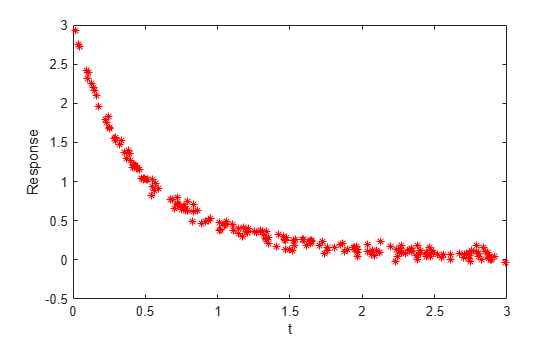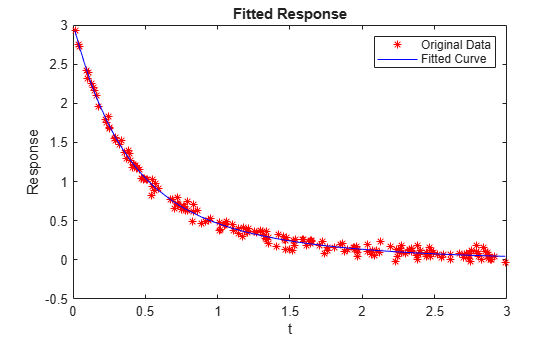# Nonlinear Least-Squares, Problem-Based

This example shows how to perform nonlinear least-squares curve fitting using the Problem-Based Optimization Workflow.

### Model

The model equation for this problem is

`$y\left(t\right)={A}_{1}\mathrm{exp}\left({r}_{1}t\right)+{A}_{2}\mathrm{exp}\left({r}_{2}t\right),$`

where ${A}_{1}$, ${A}_{2}$, ${r}_{1}$, and ${r}_{2}$ are the unknown parameters, $y$ is the response, and $t$ is time. The problem requires data for times `tdata` and (noisy) response measurements `ydata`. The goal is to find the best $A$ and $r$, meaning those values that minimize

`$\sum _{t\in tdata}{\left(y\left(t\right)-ydata\right)}^{2}.$`

### Sample Data

Typically, you have data for a problem. In this case, generate artificial noisy data for the problem. Use `A = [1,2]` and `r = [-1,-3]` as the underlying values, and use 200 random values from `0` to 3 as the time data. Plot the resulting data points.

```rng default % For reproducibility A = [1,2]; r = [-1,-3]; tdata = 3*rand(200,1); tdata = sort(tdata); % Increasing times for easier plotting noisedata = 0.05*randn(size(tdata)); % Artificial noise ydata = A(1)*exp(r(1)*tdata) + A(2)*exp(r(2)*tdata) + noisedata; plot(tdata,ydata,'r*') xlabel 't' ylabel 'Response'```The data are noisy. Therefore, the solution probably will not match the original parameters `A` and `r` very well.

### Problem-Based Approach

To find the best-fitting parameters `A` and `r`, first define optimization variables with those names.

```A = optimvar('A',2); r = optimvar('r',2);```

Create an expression for the objective function, which is the sum of squares to minimize.

```fun = A(1)*exp(r(1)*tdata) + A(2)*exp(r(2)*tdata); obj = sum((fun - ydata).^2);```

Create an optimization problem with the objective function `obj`.

`lsqproblem = optimproblem("Objective",obj);`

For the problem-based approach, specify the initial point as a structure, with the variable names as the fields of the structure. Specify the initial `A = [1/2,3/2]` and the initial `r = [-1/2,-3/2]`.

```x0.A = [1/2,3/2]; x0.r = [-1/2,-3/2];```

Review the problem formulation.

`show(lsqproblem)`
``` OptimizationProblem : Solve for: A, r minimize : sum(arg6) where: arg5 = extraParams{3}; arg6 = (((A(1) .* exp((r(1) .* extraParams{1}))) + (A(2) .* exp((r(2) .* extraParams{2})))) - arg5).^2; extraParams ```

### Problem-Based Solution

Solve the problem.

`[sol,fval] = solve(lsqproblem,x0)`
```Solving problem using lsqnonlin. Local minimum found. Optimization completed because the size of the gradient is less than the value of the optimality tolerance. <stopping criteria details> ```
```sol = struct with fields: A: [2×1 double] r: [2×1 double] ```
```fval = 0.4724 ```

Plot the resulting solution and the original data.

```figure responsedata = evaluate(fun,sol); plot(tdata,ydata,'r*',tdata,responsedata,'b-') legend('Original Data','Fitted Curve') xlabel 't' ylabel 'Response' title("Fitted Response")```The plot shows that the fitted data matches the original noisy data fairly well.

See how closely the fitted parameters match the original parameters `A = [1,2]` and `r = [-1,-3]`.

`disp(sol.A)`
``` 1.1615 1.8629 ```
`disp(sol.r)`
``` -1.0882 -3.2256 ```

The fitted parameters are off by about 15% in `A` and 8% in `r`.

### Unsupported Functions Require `fcn2optimexpr`

If your objective function is not composed of elementary functions, you must convert the function to an optimization expression using `fcn2optimexpr`. See Convert Nonlinear Function to Optimization Expression. For the present example:

```fun = @(A,r) A(1)*exp(r(1)*tdata) + A(2)*exp(r(2)*tdata); response = fcn2optimexpr(fun,A,r); obj = sum((response - ydata).^2);```

The remainder of the steps in solving the problem are the same. The only other difference is in the plotting routine, where you call `response` instead of `fun`:

`responsedata = evaluate(response,sol);`

For the list of supported functions, see Supported Operations on Optimization Variables and Expressions.